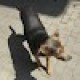# Online Solutions Development Blog   |

## Generate gradient image with php

posted by ,
Categories: PHP
Taggs , ,

There are a lot of nice effects you can get using php’s gd library. The one I decided to show now is a very simple way to render an image with a gradient between any two colors.

You will be able to use this script for a wide range of needs considering that it gives you the possibility to send as a parameter all the needed variables (initial color, final color, size and orientation).

The main idea behind this script is the fact that if you know the size of the needed output (in pixels) you can use it as the number of steps needed in making the transition between the two colors. Also, because each color can be expressed using the red, blue and green components with values between 0 and 255 you can get the arithmetic progression’s common difference by dividing the difference between each colors’ components to the number of steps (-1 for the initial step).

This way if the initial color has the red component 10 and the final color 50 and you need a 5 pixels image the red values for the steps will be 10, 20, 30, 40 and 50.

So at each step we will use something like this:

```\$color_red = floor(\$step * (\$final_color_red-\$initial_color_red) / \$image_size)+\$initial_color_red;
```

In order to assign the colors to the gd resource we can use the imagecolorallocate function this way:

```\$color = imagecolorallocate(\$image, \$color_red, \$color_green, \$color_blue);
```

Now that we have the color for the current step we need to draw a line using it, like this:

```imageline(\$image, 0, \$step, \$image_width, \$step, \$color);
```

That’s all the theory you need to understand this script.

To use this script you can call it in its default configuration like this http://localhost/gradient.php and you will get a picture like this one:

A custom way to use this script would be like this: http://localhost/gradient.php?width=300&height=200&from_r=0&from_g=255&from_b=0&to_r=0&to_g=0&to_b=255 and you will get a 300×200 image with a gradient between green and blue that will look like this:

That’s all about this script, I hope that you will like it and most of all find it useful. If you have any questions or comments please feel free to use the form below.

### 3 Responses to Generate gradient image with php

1.Reply

Thank you 🙂

2.Reply
Eder

Great script!

•Reply